# Balbharati solutions for Mathematics 2 Geometry 9th Standard Maharashtra State Board chapter 8 - Trigonometry [Latest edition]

#### Chapters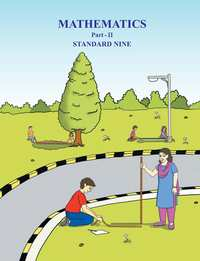## Chapter 8: Trigonometry

Practice Set 8.1Practice Set 8.2Problem Set 8
Practice Set 8.1 [Page 104]

### Balbharati solutions for Mathematics 2 Geometry 9th Standard Maharashtra State Board Chapter 8 Trigonometry Practice Set 8.1 [Page 104]

Practice Set 8.1 | Q 1 | Page 104

In the given Fig, angle R is the right angle of triangle PQR. Write the following ratios.
(i) sin P (ii) cos Q (iii) tan P (iv) tan Q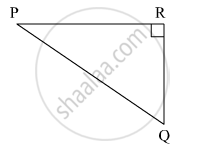Practice Set 8.1 | Q 2 | Page 104

In the right angled triangle XYZ, angleXYZ = 90° and a, b, c are the lengths of the sides as shown in the figure. Write the following ratios,
(i) sin X (ii) tan Z (iii) cos X (iv) tan X.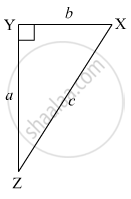Practice Set 8.1 | Q 3 | Page 104
In right angled triangle LMN, angle LMN = 90°, angle L = 50° and ∠ N = 40°, write the following ratios.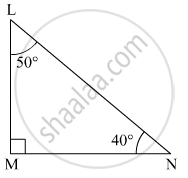(i) sin 50° (ii) cos 50° (iii) tan 40° (iv) cos 40°
Practice Set 8.1 | Q 4 | Page 104

In the given figure, angle PQR = 90° , angle PQS = 90° , angle PRQ = α  and angle QPS = θ   Write the following trigonometric ratios.
(i) sin α, cos α  , tan α
(ii) sin θ , cos θ , tan θ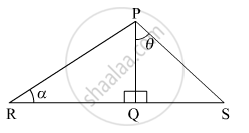Practice Set 8.2 [Page 112]

### Balbharati solutions for Mathematics 2 Geometry 9th Standard Maharashtra State Board Chapter 8 Trigonometry Practice Set 8.2 [Page 112]

Practice Set 8.2 | Q 1 | Page 112

In the following table, a ratio is given in each column. Find the remaining two ratios in the column and complete the table.

 sin θ 11/61 1/2 3/5 Cos θ 35/37 1/sqrt3 tan θ 1 21/20 8/15 1/[2sqrt2]
Practice Set 8.2 | Q 2.1 | Page 112

Find the values of

5 sin 30​° + 3 tan 45°

Practice Set 8.2 | Q 2.2 | Page 112

Find the values of

4/5 tan60° + 3sin2 60°

Practice Set 8.2 | Q 2.3 | Page 112

Find the values of

2sin 30° + cos 0° + 3sin 90°

Practice Set 8.2 | Q 2.4 | Page 112

Find the values of

 [tan 60°]/ [ sin 60° + cos 60°]

Practice Set 8.2 | Q 2.5 | Page 112

Find the values of

cos245 °+ sin2 30°

Practice Set 8.2 | Q 2.6 | Page 112

Find the values of

cos 60°× cos 30° + sin 60°× sin 30°

Practice Set 8.2 | Q 3 | Page 112

If sin θ = 4/5 then find cos θ

Practice Set 8.2 | Q 4 | Page 112

If cos θ = 15/17 then find sin θ

Problem Set 8 [Page 113]

### Balbharati solutions for Mathematics 2 Geometry 9th Standard Maharashtra State Board Chapter 8 Trigonometry Problem Set 8 [Page 113]

Problem Set 8 | Q 1.1 | Page 113

Choose the correct alternative answer for following multiple choice question.

Which of the following statements is true ?

• sin θ = cos (90 - θ )

• cos θ = tan ( 90 - θ )

• sin θ = tan (90 - θ )

• tan θ = tan (90 - θ )

Problem Set 8 | Q 1.2 | Page 113

Choose the correct alternative answer for following multiple choice question.

Which of the following is the value of sin 90° ?

• sqrt 3/2

• 0

• 1/2

• 1

Problem Set 8 | Q 1.3 | Page 113

Choose the correct alternative answer for following multiple choice question.

2 tan 45° + cos 45° - sin 45° = ?

• 0

• 1

• 2

• 3

Problem Set 8 | Q 1.4 | Page 113

Choose the correct alternative answer for following multiple choice question.

[cos 28°]/[sin 62°]

• 2

• -1

• 0

• 1

Problem Set 8 | Q 2 | Page 113

In right angled Δ TSU, TS = 5, ∠S = 90°, SU = 12 then find sin T, cos T, tan T. Similarly find sin U, cos U, tan U.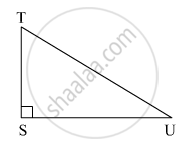Problem Set 8 | Q 3 | Page 113

In right angled ΔYXZ, ∠ X = 90°, XZ = 8 cm, YZ = 17 cm, find sin Y, cos Y, tan Y, sin Z, cos Z, tan Z.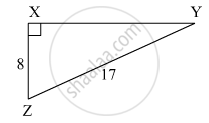Problem Set 8 | Q 4 | Page 113

In right angled Δ LMN , if ∠ N =θ , ∠M = 90°, cos θ =24/25 , find sin θ and tan θ Similarly, find  ( sin2θ) and ( cos2θ).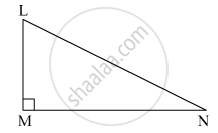Problem Set 8 | Q 5.1 | Page 113

Fill in the blank.
sin20  =  cos ________ °

Problem Set 8 | Q 5.2 | Page 113

Fill in the blank.

tan 30° x tan ______°  = 1

Problem Set 8 | Q 5.3 | Page 113

Fill in the blank.

cos 40° = sin ______°

## Chapter 8: Trigonometry

Practice Set 8.1Practice Set 8.2Problem Set 8## Balbharati solutions for Mathematics 2 Geometry 9th Standard Maharashtra State Board chapter 8 - Trigonometry

Balbharati solutions for Mathematics 2 Geometry 9th Standard Maharashtra State Board chapter 8 (Trigonometry) include all questions with solution and detail explanation. This will clear students doubts about any question and improve application skills while preparing for board exams. The detailed, step-by-step solutions will help you understand the concepts better and clear your confusions, if any. Shaalaa.com has the Maharashtra State Board Mathematics 2 Geometry 9th Standard Maharashtra State Board solutions in a manner that help students grasp basic concepts better and faster.

Further, we at Shaalaa.com provide such solutions so that students can prepare for written exams. Balbharati textbook solutions can be a core help for self-study and acts as a perfect self-help guidance for students.

Concepts covered in Mathematics 2 Geometry 9th Standard Maharashtra State Board chapter 8 Trigonometry are Trigonometry, Terms related to right angled triangle, Important Equation in Trigonometry, Method of Using Trigonometric Table, Trigonometric Ratios and Its Reciprocal, Trigonometric Ratios and Its Reciprocal.

Using Balbharati 9th Standard solutions Trigonometry exercise by students are an easy way to prepare for the exams, as they involve solutions arranged chapter-wise also page wise. The questions involved in Balbharati Solutions are important questions that can be asked in the final exam. Maximum students of Maharashtra State Board 9th Standard prefer Balbharati Textbook Solutions to score more in exam.

Get the free view of chapter 8 Trigonometry 9th Standard extra questions for Mathematics 2 Geometry 9th Standard Maharashtra State Board and can use Shaalaa.com to keep it handy for your exam preparation